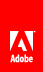# Performing complex 3D transformations

Flash Player 10 and later, Adobe AIR 1.5 and later

The Matrix3D class lets you transform 3D points within a coordinate space or map 3D points from one coordinate space to another.

You don't need to understand matrix mathematics to use the Matrix3D class. Most of the common transformation operations can be handled using the methods of the class. You don't have to worry about explicitly setting or calculating the values of each element in the matrix.

After you set the z property of a display object to a numeric value, you can retrieve the object’s transformation matrix using the Matrix3D property of the display object’s Transform object:

`var leafMatrix:Matrix3D = this.transform.matrix3D;`

You can use the methods of the Matrix3D object to perform translation, rotation, scaling, and perspective projection on the display object.

Use the Vector3D class with its x , y , and z properties for managing 3D points. It can also represent a spatial vector in physics, which has a direction and a magnitude. The methods of the Vector3D class let you perform common calculations with spatial vectors, such as addition, dot product, and cross product calculations.

Note: The Vector3D class is not related to the ActionScript Vector class. The Vector3D class contains properties and methods for defining and manipulating 3D points, while the Vector class supports arrays of typed objects.

## Creating Matrix3D objects

There are three main ways of creating or retrieving Matrix3D objects:

• Use the Matrix3D() constructor method to instantiate a new matrix. The Matrix3D() constructor takes a Vector object containing 16 numeric values and places each value into a cell of the matrix. For example:

`var rotateMatrix:Matrix3D = new Matrix3D(1,0,0,1, 0,1,0,1, 0,0,1,1, 0,0,0,1);`
• Set the value the z property of a display object. Then retrieve the transformation matrix from the transform.matrix3D property of that object.

• Retrieve the Matrix3D object that controls the display of 3D objects on the stage by calling the perspectiveProjection.toMatrix3D() method on the root display object.

## Applying multiple 3D transformations

You can apply many 3D transformations at once using a Matrix3D object. For example if you wanted to rotate, scale, and then move a cube, you could apply three separate transformations to each point of the cube. However it is much more efficient to precalculate multiple transformations in one Matrix3D object and then perform one matrix transformation on each of the points.

Note: The order in which matrix transformations are applied is important. Matrix calculations are not commutative. For example, applying a rotation followed by a translation gives a different result than applying the same translation followed by the same rotation.

The following example shows two ways of performing multiple 3D transformations.

```package {
import flash.display.Sprite;
import flash.display.Shape;
import flash.display.Graphics;
import flash.geom.*;

public class Matrix3DTransformsExample extends Sprite
{
private var rect1:Shape;
private var rect2:Shape;

public function Matrix3DTransformsExample():void
{
var pp:PerspectiveProjection = this.transform.perspectiveProjection;
pp.projectionCenter = new Point(275,200);
this.transform.perspectiveProjection = pp;

rect1 = new Shape();
rect1.x = -70;
rect1.y = -40;
rect1.z = 0;
rect1.graphics.beginFill(0xFF8800);
rect1.graphics.drawRect(0,0,50,80);
rect1.graphics.endFill();

rect2 = new Shape();
rect2.x = 20;
rect2.y = -40;
rect2.z = 0;
rect2.graphics.beginFill(0xFF0088);
rect2.graphics.drawRect(0,0,50,80);
rect2.graphics.endFill();

doTransforms();
}

private function doTransforms():void
{
rect1.rotationX = 15;
rect1.scaleX = 1.2;
rect1.x += 100;
rect1.y += 50;
rect1.rotationZ = 10;

var matrix:Matrix3D = rect2.transform.matrix3D;
matrix.appendRotation(15, Vector3D.X_AXIS);
matrix.appendScale(1.2, 1, 1);
matrix.appendTranslation(100, 50, 0);
matrix.appendRotation(10, Vector3D.Z_AXIS);
rect2.transform.matrix3D = matrix;
}
}
}```

In the doTransforms() method the first block of code uses the DisplayObject properties to change the rotation, scaling, and position of a rectangle shape. The second block of code uses the methods of the Matrix3D class to do the same transformations.

The main advantage of using the Matrix3D methods is that all of the calculations are performed in the matrix first,. Then they are applied to the display object only once, when its transform.matrix3D property is set. Setting DisplayObject properties make the source code a bit simpler to read. However each time a rotation or scaling property is set, it causes multiple calculations and changes multiple display object properties.

If your code will apply the same complex transformations to display objects more than once, save the Matrix3D object as a variable and then reapply it over and over.

## Using Matrix3D objects for reordering display

As mentioned previously, the layering order of display objects in the display list determines the display layering order, regardless of their relative z-axes. If your animation transforms the properties of display objects into an order that differs from the display list order, the viewer might see display object layering that does not correspond to the z-axis layering. So, an object that should appear further away from the viewer might appear in front of an object that is closer to the viewer.

To ensure that the layering of 3D display objects corresponds to the relative depths of the objects, use an approach like the following:

1. Use the getRelativeMatrix3D() method of the Transform object to get the relative z-axes of the child 3D display objects.

2. Use the removeChild() method to remove the objects from the display list.

3. Sort the display objects based on their relative z-axis values.

4. Use the addChild() method to add the children back to the display list in reverse order.

This reordering ensures that your objects display in accordance with their relative z-axes.

The following code enforces the correct display of the six faces of a 3D box. It reorders the faces of the box after rotations have been applied to the it:

```public var faces:Array; . . .

public function ReorderChildren()
{
for(var ind:uint = 0; ind < 6; ind++)
{
faces[ind].z = faces[ind].child.transform.getRelativeMatrix3D(root).position.z;
this.removeChild(faces[ind].child);
}
faces.sortOn("z", Array.NUMERIC | Array.DESCENDING);
for (ind = 0; ind < 6; ind++)
{